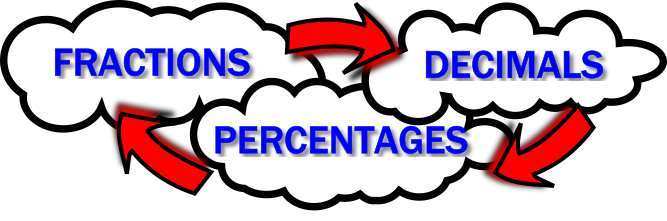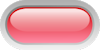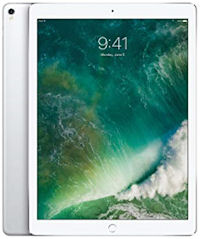#Fraction Decimal Percentage$$\frac12$$ $$0.5$$ $$50$$%$$\frac34$$ $$0.75$$ $$75$$%$$\frac14$$ $$0.25$$ $$25$$%$$\frac15$$ $$0.2$$ $$20$$%$$\frac35$$ $$0.6$$ $$60$$%$$\frac{11}{20}$$ $$0.55$$ $$55$$%$$\frac{7}{10}$$ $$0.7$$ $$70$$%$$\frac{1}{25}$$ $$0.04$$ $$4$$%$$\frac{3}{10}$$ $$0.3$$ $$30$$%$$\frac{3}{100}$$ $$0.03$$ $$3$$%$$\frac{17}{20}$$ $$0.85$$ $$85$$%$$\frac{1}{3}$$ $$0.\dot3$$ $$33\frac13$$%$$\frac{2}{3}$$ $$0.\dot6$$ $$66\frac23$$%$$\frac{1}{8}$$ $$0.125$$ $$12\frac12$$%$$\frac{3}{8}$$ $$0.375$$ $$37\frac12$$%$$\frac{7}{8}$$ $$0.875$$ $$87\frac12$$%$$\frac{5}{8}$$ $$0.625$$ $$62\frac12$$%$$\frac{1}{40}$$ $$0.025$$ $$2\frac12$$%$$\frac{17}{40}$$ $$0.425$$ $$42\frac12$$%$$\frac{7}{200}$$ $$0.035$$ $$3\frac12$$%$$\frac{7}{150}$$ $$0.04\dot6$$ $$4\frac23$$%## Copy and complete this table.

Teacher! You can customise this starter to suit your pupils. Click here to show all possible rows in the table above then click on the red buttons to hide the rows you don't want.

A short slide presentation is available to revise the operations needed to convert between fractions, decimals and percentages.

## A Mathematics Lesson Starter Of The Day

Share

How did you use this starter? Can you suggest how teachers could present or develop this resource? Do you have any comments? It is always useful to receive feedback and helps make this free resource even more useful for Maths teachers anywhere in the world.

If you don't have the time to provide feedback we'd really appreciate it if you could give this page a score! We are constantly improving and adding to these starters so it would be really helpful to know which ones are most useful. Simply click on a button below:

Excellent, I would like to see more like this
Good, achieved the results I required
Satisfactory
Didn't really capture the interest of the students
Not for me! I wouldn't use this type of activity.

This starter has scored a mean of 2.9 out of 5 based on 328 votes.

Previous Day | This starter is for 27 July | Next Day

Your access to the majority of the Transum resources continues to be free but you can help support the continued growth of the website by doing your Amazon shopping using the links on this page. Below is an Amazon search box and some items chosen and recommended by Transum Mathematics to get you started.

## Texas Instruments Nspire Calculator

This handheld device and companion software are designed to generate opportunities for classroom exploration and to promote greater understanding of core concepts in the mathematics and science classroom. TI-Nspire technology has been developed through sound classroom research which shows that "linked multiple representation are crucial in development of conceptual understanding and it is feasible only through use of a technology such as TI-Nspire, which provides simultaneous, dynamically linked representations of graphs, equations, data, and verbal explanations, such that a change in one representation is immediately reflected in the others.

For the young people in your life it is a great investment. Bought as a gift for a special occasion but useful for many years to come as the young person turns into an A-level candidate then works their way through university. more...## Apple iPad Pro

The analytics show that more and more people are accessing Transum Mathematics via an iPad as it is so portable and responsive. The iPad has so many other uses in addition to solving Transum's puzzles and challenges and it would make an excellent gift for anyone.

The redesigned Retina display is as stunning to look at as it is to touch. It all comes with iOS, the world's most advanced mobile operating system. iPad Pro. Everything you want modern computing to be. more...

Before giving an iPad as a Christmas gift you could add a link to iPad Maths to the home screen.

Click the images above to see all the details of these items and to buy them online.Teacher, do your students have access to computers?Do they have iPads or Laptops in Lessons? Whether your students each have a TabletPC, a Surface or a Mac, this activity lends itself to eLearning (Engaged Learning).Here a concise URL for a version of this page without the comments.

Transum.org/go/?Start=July27

Here is the URL which will take them to a related student activity.

Transum.org/go/?to=fdp## Curriculum Reference

See the National Curriculum page for links to related online activities and resources.For All: